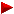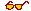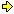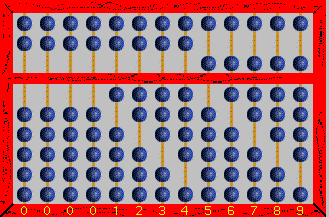HOME plateWON | World!OfNumbersThe Nine Digits Page 1 with some Ten Digits (pandigital) exceptionsPage 2Page 3Page 4Page 5Page 6Page 7

When I use the term ninedigital in these articles I always refer to a strictly zeroless pandigital (digits from 1 to 9 each appearing just once).

First Page

Topic 1.9
( Source : Recreations in the Theory of Numbers )

[ Chapter VIII : Digits - and the magic of 9 ]
[ I found the same exposé in Shakuntala Devi's book “Figuring : The Joy of Numbers” ]

"The following curious table shows how to arrange the 9 digits so that the product
of 2 groups is equal to a number represented by the remaining digits."

12 x 483 = 5796
42 x 138 = 5796
18 x 297 = 5346
27 x 198 = 5346
39 x 186 = 7254
48 x 159 = 7632
28 x 157 = 4396
4 x 1738 = 6952
4 x 1963 = 7852

"The product of 51249876 and 3 (all the digits used once only) is 153749628,
which again contains all 9 digits used once."

16583742 x 9 = 149253678
32547891 x 6 = 195287346

[ Chapter XIV : The eternal triangle ]
"There are many Pythagorean triangles whose area utilizes 9 different digits.
Using generators 149 and 58, the area is 162789354 ;
using 224 and 153, the area is 917358624."

[ Chapter XV : On the square ]
"The following table shows the difference of two squares equal to a number containing
all the nine digits used only once."
Readers should recall that x2y2 = (x + y) x (x – y), hence the second expression !

1111322002 = 11313 x 10913 = 123458769
3111122002 = 31311 x 30911 = 967854321
1111722002 = 11317 x 10917 = 123547689
11356220002 = 13356 x 9356 = 124958736
12695260172 = 18712 x 6.678 = 124958736
162602118082 = 28068 x 4452 = 124958736
1237223002 = 12672 x 12072 = 152976384

"Express the numbers whose digits are in regularly ascending or descending order
as the difference of two squares."

 123456789 Factorization = 32 x 3607 x 3803 = 617283952 – 617283942 = 205761332 – 205761302 = 68587152 – 68587062 = 189172 – 153102 = 181332 – 143302 = 111152 – 2942 987654321 Factorization = 32 x 172 x 379721 = 4938271612 – 4938271602 = 1646090552 – 1646090522 = 548696892 – 548696802 = 290486652 – 290486482 = 96829112 – 96828602 = 32277052 – 32275522 = 17088892 – 17086002 = 5700152 – 5691482 = 1911612 – 1885602

Topic 1.8
Martin Gardner introduced the following puzzle
( Source : Further Mathematical Diversions )

[ Chapter 15 : Nine Problems : nr.8 : NINE TO ONE EQUALS 100 ]
"An old numerical problem that keeps reappearing in puzzle books as though it had never been analyzed before is the problem of inserting mathematical signs wherever one likes between the digits 1, 2, 3, 4, 5, 6, 7, 8, 9 to make the expression equal 100. The digits must remain in the same sequence. There are many hundreds of solutions, the easiest to find perhaps being "

1 + 2 + 3 + 4 + 5 + 6 + 7 + ( 8 x 9 ) = 100

"The problem becomes more of a challenge if the mathematical signs are limited to plus and minus. Here again there are many solutions, for example"

1 + 2 + 345 + 678 + 9 = 100
12 + 34 + 5 + 67 + 8 + 9 = 100
1234567 + 89 = 100
123 + 45 + 6789 = 100
123 + 4567 + 89 = 100
1234567 + 89 = 100

"In view of the popularity of this problem it is surprising that so little effort seems to have been spent on the problem in reverse form. That is, take the digits in descending order, 9 through 1, and form an expression equal to 100 by inserting the smallest possible number of plus and minus signs.

Answer : To form an expression equal to 100, four plus and minus signs can be inserted between the digits, taken in reverse order, as follows ¬"

9876 + 54 + 3 + 21 = 100

"There is no other solution with as few as four signs !"I found some online source dealing also with these 'cryptarithms'.
Check out for more variations on the theme :
Fun with digits
Expression = 100

[ May 10, 2002 ]
Barry Sanders (email) asked himself if there is a solution using each of the digits 1 to 9 once, but
they don't have to be in consecutive order, in combination with only the PLUS sign ( + ) sums to 100 ?
Is there an answer ? Is it unique ?
Is there a program that would show all possibilities ?

Not able to answer Barry satisfactorily he threw the topic into
a discussion newsgroup alt.math where very soon afterwards
Terry Moriarty (email) from Northern Ireland gave the following interesting reply :

Consider the following :
The sum of all numbers 1-9 is 45.
Since this is too low (to reach 100) you must use some 2 figure numbers (23, 34, etc.)
but *all* of these give you an addition of a multiple of 9
for instance use '12' instead of '1+2'
( creates an additional 9 since you have to remove the 1 and 2 )
your total is now 45 - 1 - 2 + 12 = 54.
No matter what doubles you choose, they will always add some multiple of 9.

Assume that the high part of your double is 'a' and the low part is 'b'
(10a + b) - a - b = 9a
For you to succeed you need to add 55 (to reach 100) to your original total.You can't do this with multiples of 9.

Topic 1.7
Variations on a theme
by the same Martin Gardner ( Source : Puzzles from other Worlds )
playing with The Number of the Beast

"Three plus signs can be inserted within the sequence 123456789 to make a sum of 666"

123 + 456 + 78 + 9 = 666

"If minus signs are also allowed (except that a minus sign is not permitted in front of the sequence), what is the only other way to obtain 666 with just three signs ? The sum cannot be achieved with fewer signs."

Answer : 1234567 + 89 = 666

"Find the only way to insert four signs (each may be plus or minus) inside 987654321 to make a sum of 666. Again, there is no solution with fewer signs."

Answer : 9 + 87 + 6 + 543 + 21 = 666

"A common interpretation among today's fundamentalists is that 666 represents a falling short of 777, which is taken to be a symbol of perfection. I believe there is no way to insert any number of plus and minus signs in 123456789 to get a sum of 777, and just one way to do it with 987654321. How ? "

Answer : 98 + 7 + 6543 + 21 = 777

 666 has eight solutions for the ascending sequence ¬ – 1 + 2 – 3 + 4 – 5 + 678 – 9 + 1 – 2 – 3 – 4 + 5 + 678 – 9 + 1 + 2 + 3 – 4 – 5 + 678 – 9 + 1 – 23 – 4 + 5 + 678 + 9 + 1 + 2 + 3 + 4 + 567 + 89 + 1 + 23 – 45 + 678 + 9 + 123 + 456 + 78 + 9 + 1234 – 567 + 8 – 9 And five solutions for the descending ¬ – 9 + 8 + 7 + 654 + 3 + 2 + 1 + 9 – 8 + 7 + 654 + 3 + 2 – 1 + 9 + 8 – 7 + 654 + 3 – 2 + 1 + 9 – 8 – 7 + 654 – 3 + 21 + 9 + 87 + 6 + 543 + 21

 777 has two solutions for the ascending sequence ¬ – 12 – 3 + 4 + 5 – 6 + 789 – 12 + 3 – 4 – 5 + 6 + 789 And two for the descending ¬ – 9 – 8 + 765 – 4 + 32 + 1 + 98 + 7 + 654 – 3 + 21

Topic 1.6   [ January 10, 2001 ]
A nice relation between these two repdigits from topic 1.7
by Patrick De Geest

There exists a nice relation between 666 and 777 although a rather convoluted one.
Replace the three 6 digits with the 6th prime or 13.
The juxtaposed digits from 666 become thus (13)(13)(13) and we can make the following fraction ¬
131313
13 * 13
= 777
Another argument that the number of the beast is closely related to 13.
Too bad for those who are a bit triskaidekaphobic and/or suffering from aibohphobia .

Topic 1.5
Here is a beautiful numerical expansion. Guess where it leads us to ?

Websource : Number 8
Booksource : “Numbers: Facts, Figures and Fiction” by Richard Phillips
1 x 8 + 1 = 9
12 x 8 + 2 = 98
123 x 8 + 3 = 987
1234 x 8 + 4 = 9876
12345 x 8 + 5 = 98765
123456 x 8 + 6 = 987654
1234567 x 8 + 7 = 9876543
12345678 x 8 + 8 = 98765432
123456789 x 8 + 9 = 987654321

Topic 1.4
Ever wondered how the ancient Chinese represented 123456789
on the Abacus ?

Click on the image to get an introduction to the abacus.There are more sites that explains the beads and rods of the abacus.

Topic 1.3
Nine Digits forming Unity 1

This comes straight out of the archive of the newsgroup rec.puzzles

==> arithmetic/digits/equations/123456789.p <==

In how many ways can "" be replaced with "+", "–", or "" (concatenate) in
•1•2•3•4•5•6•7•8•9=1 to form a correct equation ?

==> arithmetic/digits/equations/123456789.s <==

 ``` 1-2 3+4 5+6 7-8 9 = 1 +1-2 3+4 5+6 7-8 9 = 1 1+2 3+4-5+6 7-8 9 = 1 +1+2 3+4-5+6 7-8 9 = 1 -1+2 3-4+5+6 7-8 9 = 1 1+2 3-4 5-6 7+8 9 = 1 +1+2 3-4 5-6 7+8 9 = 1 1-2 3-4+5-6 7+8 9 = 1 +1-2 3-4+5-6 7+8 9 = 1 1-2-3-4 5+6 7-8-9 = 1 +1-2-3-4 5+6 7-8-9 = 1 1+2-3 4+5 6-7-8-9 = 1 +1+2-3 4+5 6-7-8-9 = 1 -1+2 3+4+5-6-7-8-9 = 1 -1 2+3 4-5-6+7-8-9 = 1 1+2+3+4-5+6+7-8-9 = 1 +1+2+3+4-5+6+7-8-9 = 1 -1+2+3-4+5+6+7-8-9 = 1 1-2-3+4+5+6+7-8-9 = 1 +1-2-3+4+5+6+7-8-9 = 1 1+2 3+4 5-6 7+8-9 = 1 +1+2 3+4 5-6 7+8-9 = 1 1+2 3-4-5-6-7+8-9 = 1 ``` ```+1+2 3-4-5-6-7+8-9 = 1 1+2+3+4+5-6-7+8-9 = 1 +1+2+3+4+5-6-7+8-9 = 1 -1+2+3+4-5+6-7+8-9 = 1 1-2+3-4+5+6-7+8-9 = 1 +1-2+3-4+5+6-7+8-9 = 1 -1-2-3+4+5+6-7+8-9 = 1 1-2+3+4-5-6+7+8-9 = 1 +1-2+3+4-5-6+7+8-9 = 1 1+2-3-4+5-6+7+8-9 = 1 +1+2-3-4+5-6+7+8-9 = 1 -1-2+3-4+5-6+7+8-9 = 1 -1+2-3-4-5+6+7+8-9 = 1 -1+2 3+4 5-6 7-8+9 = 1 1-2 3-4 5+6 7-8+9 = 1 +1-2 3-4 5+6 7-8+9 = 1 -1+2 3-4-5-6-7-8+9 = 1 -1+2+3+4+5-6-7-8+9 = 1 1-2+3+4-5+6-7-8+9 = 1 +1-2+3+4-5+6-7-8+9 = 1 1+2-3-4+5+6-7-8+9 = 1 +1+2-3-4+5+6-7-8+9 = 1 -1-2+3-4+5+6-7-8+9 = 1 ``` ``` 1+2-3+4-5-6+7-8+9 = 1 +1+2-3+4-5-6+7-8+9 = 1 -1-2+3+4-5-6+7-8+9 = 1 -1+2-3-4+5-6+7-8+9 = 1 1-2-3-4-5+6+7-8+9 = 1 +1-2-3-4-5+6+7-8+9 = 1 1-2 3+4+5+6+7-8+9 = 1 +1-2 3+4+5+6+7-8+9 = 1 1+2+3+4 5-6 7+8+9 = 1 +1+2+3+4 5-6 7+8+9 = 1 1 2+3 4+5-6 7+8+9 = 1 +1 2+3 4+5-6 7+8+9 = 1 1+2+3-4-5-6-7+8+9 = 1 +1+2+3-4-5-6-7+8+9 = 1 -1+2-3+4-5-6-7+8+9 = 1 1-2-3-4+5-6-7+8+9 = 1 +1-2-3-4+5-6-7+8+9 = 1 -1-2-3-4-5+6-7+8+9 = 1 -1-2 3+4+5+6-7+8+9 = 1 1-2+3 4-5 6+7+8+9 = 1 +1-2+3 4-5 6+7+8+9 = 1 1 2-3 4+5-6+7+8+9 = 1 +1 2-3 4+5-6+7+8+9 = 1 ```

Total solutions = 69
(26 of which have a leading "+", which is redundant)
69/19683 = 0.35 %

Topic 1.2
Commissioned for BBC Education as part of the Count Me In Numeracy Site

https://nrich.maths.org/2007
The sum of 1 + 2 + 3 + 4 + 5 + 6 + 7 + 8 + 9 = 45
Multiply 123456789 by 2 and you get 246913578, the sum of which is 45
Divide 123456789 by 2 and you get 61728394.5, the sum of which is 45

Topic 1.1

This is another puzzle found on the web dealing with the digits one trought nine.
For instance https://donsteward.blogspot.com/2012/04/olympic-rings.html

Given the five olympic rings how can the digits one through nine be placed within the nine regions
(five non-overlapping ring regions and four overlapping regions shared between two rings)
so that each ring contains the same total ?

Or alternatively, using the labels A through I for the regions, how can the numbers one through nine
be assigned to the variables such that :

A + B = B + C + D = D + E + F = F + G + H = H + I

Can you solve it ?
SPOILER : it is hidden somewhere in the source code of this page !

For a logical and detailed approach other than brute force consult
Ken Duisenberg's Puzzle-Of-The-Week Archive - Olympic Ring Addition.

For reference goals and easy searching I list here all the nine- & pandigitals implicitly displayed in these topics.

Topic 1.9 → 124835796, 421385796, 182975346, 271985346, 391867254, 481597632, 281574396, 417386952, 419637852, 512498763, 165837429, 325478916

Topic 1.8 → 123456789, 987654321

Topic 1.7 → 123456789, 987654321

Topic 1.5 → 123456789, 987654321

More Integer Sequences from Sloane's OEIS Database

1. A034277 Decimal part of a(n)^(1/2) starts with a 'nine digits' anagram. - Patrick De Geest
2. A034278 Decimal part of a(n)^(1/3) starts with a 'nine digits' anagram. - Patrick De Geest
3. A034279 Decimal part of a(n)^(1/4) starts with a 'nine digits' anagram. - Patrick De Geest
4. A034280 Decimal part of a(n)^(1/5) starts with a 'nine digits' anagram. - Patrick De Geest
5. A034281 Decimal part of a(n)^(1/6) starts with a 'nine digits' anagram. - Patrick De Geest
6. A034282 Decimal part of a(n)^(1/7) starts with a 'nine digits' anagram. - Patrick De Geest
7. A034283 Decimal part of a(n)^(1/8) starts with a 'nine digits' anagram. - Patrick De Geest
8. A034284 Decimal part of a(n)^(1/9) starts with a 'nine digits' anagram. - Patrick De Geest
9. A034285 Decimal part of a(n)^(1/10) starts with a 'nine digits' anagram. - Patrick De Geest
10. A034286 Decimal part of a(n)^(1/11) starts with a 'nine digits' anagram. - Patrick De Geest
11. A034306 Fibonacci iteration F(1,palindrome a(n)) leads to a 'nine digits' anagram. - Patrick De Geest
12. A034587 Fibonacci iteration F(1,a(n)) leads to a 'nine digits' anagram. - Patrick De Geest
13. A034588 Fibonacci iteration F(1,prime p(n)) leads to a 'nine digits' anagram. - Patrick De Geest
14. A034589 Fibonacci iteration F(1,lucky number a(n)) leads to a 'nine digits' anagram. - Patrick De Geest
15. A034590 Decimal part of square root of 'nine digits anagram' starts with a 'nine digits anagram'. - Patrick De Geest
16. A035136 Decimal part of a(n)^(1/n) starts with a 'nine digits' anagram. - Patrick De Geest
17. A035304 Decimal part of a(n)^(1/n) starts with a pandigital anagram (digits 0 through 9 in some order). - Erich Friedman
18. A036744 Penholodigital squares: includes each digit once. - dww (wilson)
19. A036745 Holodigital squares: includes each digit once. - dww (wilson)
20. A039667 Pandigital sums: n such that n+x=y with x=2n and y=3n. - Felice Russo
21. A046043 Autobiographical (or curious) numbers: n = x0 x1 x2...x9 such that xi is the number of digits equal to i in n. - Robert Leduc
22. A049442 Sum of first n consecutive prime numbers is pandigital (includes all 10 digits exactly once). - G. L. Honaker, Jr.
23. A049443 Sum of consecutive primes to p is pandigital (includes all 10 digits exactly once). - G. L. Honaker, Jr.
24. A049446 Pandigital sums associated with A049442. - G. L. Honaker, Jr.
25. A050278 Pandigital numbers: numbers containing the digits 0-9. - Eric W. Weisstein
26. A050288 Pandigital primes. - Eric W. Weisstein
27. A050289 Zeroless pandigital numbers: numbers containing the digits 1-9 and no 0's. - Eric W. Weisstein
28. A050290 Zeroless pandigital primes. - Eric W. Weisstein
29. A053654 Multiples of 123456789. - Klaus Strassburger
30. A054037 n^2 contains exactly 9 different digits. - Asher Auel
31. A054038 n^2 contains every digit at least once. - Asher Auel
32. A054383 Number of pandigital fractions for 1/n. - Eric W. Weisstein
33. A058760 Integers whose set of prime factors (taken with multiplicity) uses each digit exactly once (i.e. is pandigital) in some base b>1. Numbers are expressed in base 10. - G. L. Honaker, Jr. and Mike Keith
34. A058908 Six prime numbers in arithmetical progression with a common difference of 9876543210. - G. L. Honaker, Jr.
35. A058909 Integers whose set of prime factors (taken with multiplicity) uses each digit exactly once (i.e., is pandigital) in base 10. - Mike Keith and G. L. Honaker, Jr.
36. A071519 Numbers whose squares are zeroless pandigitals (i.e. use the digits 1 through 9 once). - Lekraj Beedassy
37. A074205 Smallest positive integer whose n-th power contains an equal number of each digit (0-9) when represented in base 10. - Jack Brennen

Sources

Prime Curios! - site maintained by G. L. Honaker Jr. and Chris Caldwell
3
5
89
999
3187
5077
5986
28651
32423
14368485
15618090
36543120
40578660
45269999
394521678
1123465789
1227182861
1531415939
2543568463
5897230146
10123457689
1023456987896543201
5021837752995317770489

Contributions
```

```

[TOP OF PAGE]

Patrick De Geest - Belgium- Short Bio - Some Pictures